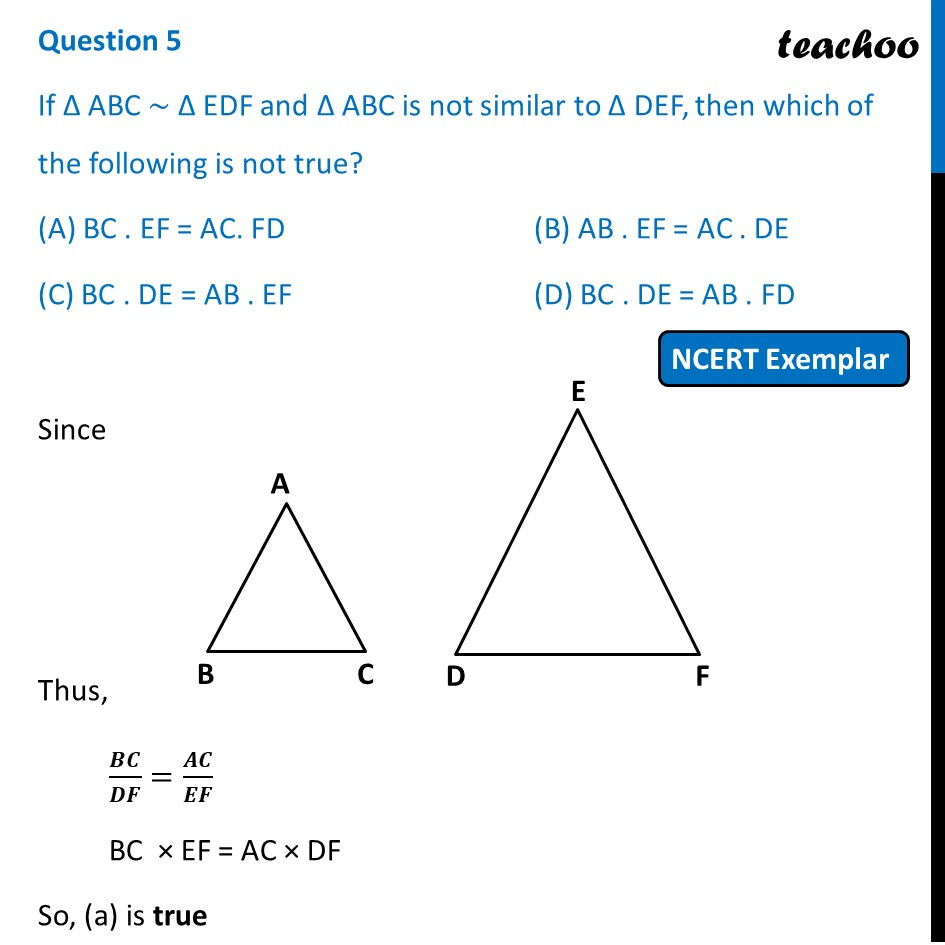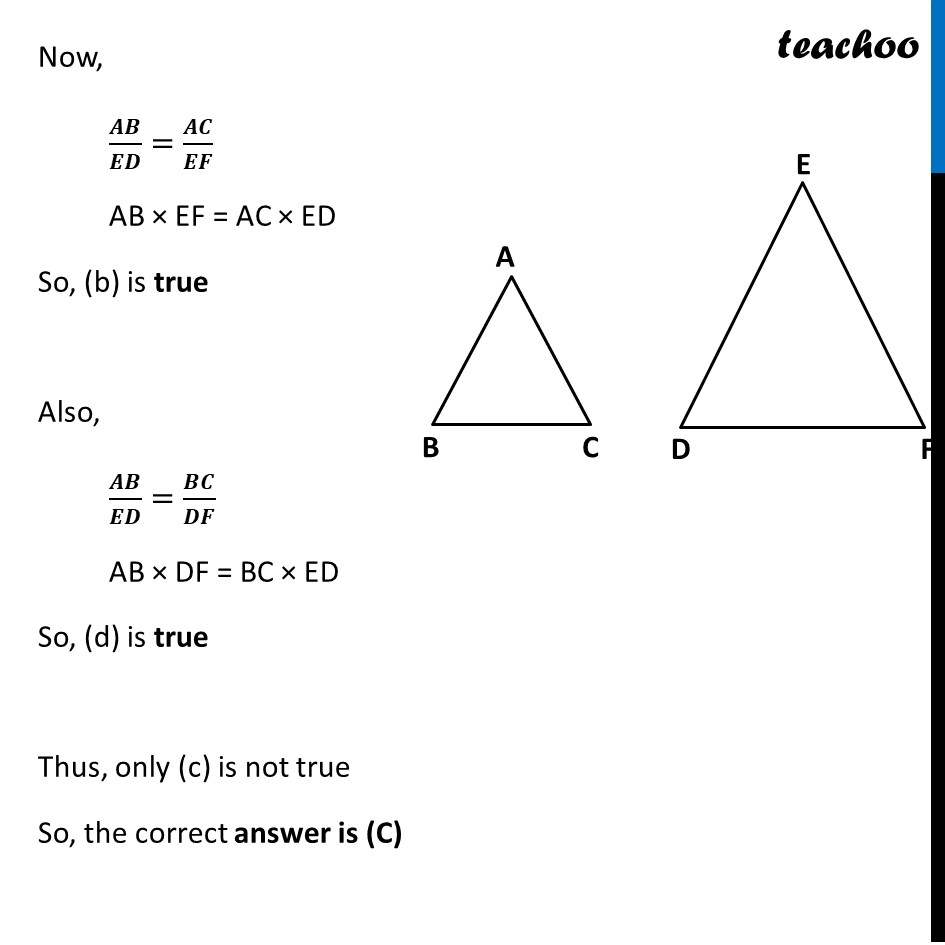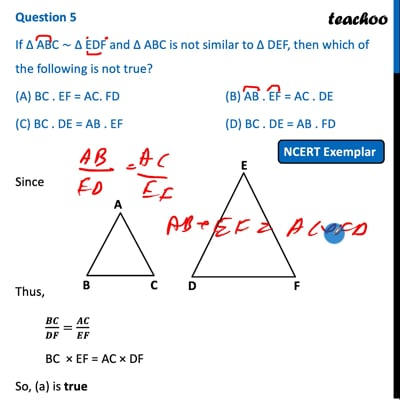NCERT Exemplar - MCQ

Chapter 6 Class 10 Triangles
Serial order wise

## (D) BC . DE = AB . FDThis video is only available for Teachoo black users

Introducing your new favourite teacher - Teachoo Black, at only ₹83 per month

### Transcript

Question 5 If ∆ ABC ~ ∆ EDF and ∆ ABC is not similar to ∆ DEF, then which of the following is not true? (A) BC . EF = AC. FD (B) AB . EF = AC . DE (C) BC . DE = AB . EF (D) BC . DE = AB . FD Since Thus, 𝑩𝑪/𝑫𝑭=𝑨𝑪/𝑬𝑭 BC × EF = AC × DF So, (a) is true So, the correct answer is (C) Now, 𝑨𝑩/𝑬𝑫=𝑨𝑪/𝑬𝑭 AB × EF = AC × ED So, (b) is true Also, 𝑨𝑩/𝑬𝑫=𝑩𝑪/𝑫𝑭 AB × DF = BC × ED So, (d) is true Thus, only (c) is not true So, the correct answer is (C)